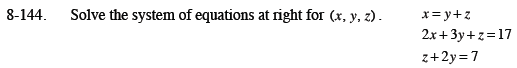### Home > CCA2 > Chapter Ch8 > Lesson 8.3.2 > Problem8-144

8-144.

Solve the system of equations below for (x, y, z). Homework Help ✎ x = y + z
2x + 3y + z = 17
z + 2y = 7Substitute y + z for x into the second equation.

Multiply the third equation by −3 then add it to the earlier equation.

Substitute 4 for y in the third equation to solve for z.

Substitute 4 for y and −1 for z in the first equation to solve for x.

Check your values for x, y, and z in the second equation.

2y + 2z + 3y + z = 17
3z + 5y = 17

3z + 5y = 17
−3z − 6y = −21
y = −4
y = 4

z + 8 = 7
z = −1

x = 4 − 1
x = 3

(3, 4, −1)